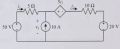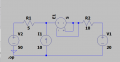# LTspice circuit problem

#### soooo

Joined Apr 19, 2021
7
Hello! I'm using LTspice and I'm not quite sure how I'm supposed to do the dependent source as it isn't dependent on a component. The circuit reference and my attempt (which turned out wrong) are attached below:Thanks in advanceLast edited:

#### crutschow

Joined Mar 14, 2008
27,915

But it don't think the above circuit can work, since the current source is multiplying its own current which would lead to infinite current..

#### soooo

Joined Apr 19, 2021
7

But it don't think the above circuit can work, since the current source is multiplying its own current which would lead to infinite current..
It's actually not the same concept, I've tried adding Bv with V=5*(I{R2}) and it also turned out to be incorrect so I'm not sure where the mistake is happening.

#### Irving

Joined Jan 30, 2016
2,177
Surely thediamond is a current controlled voltage where V= 5i. The current has to flowthrough the control path, which it cant do there as the two connections to the current control are shorted. Break the link between the twocurrent control inputs.

#### crutschow

Joined Mar 14, 2008
27,915
so I'm not sure where the mistake is happening.

#### Irving

Joined Jan 30, 2016
2,177
•soooo

#### Irving

Joined Jan 30, 2016
2,177
Nor I ! The important thing of note is that it doesn't have a control wire but uses a current value from a controlling 'source'. That 'source' must be an actual source i.e. a voltage generator (I'm guessing it could be a current source too). So if you didn't have a voltage source (in this case V2) you have to put a zero volt 'source' in series with the component you want the current derived from. Confusing huh?!

#### crutschow

Joined Mar 14, 2008
27,915
Alternately, you can use R2 for the current source.
Connect the control terminals for the voltage-controlled voltage source in your first schematic across R2.
Then calculate the proper gain for the voltage source, based upon the resistance of R2, to give you 5i2 for the overall gain.

Last edited:
•Irving

#### Irving

Joined Jan 30, 2016
2,177
You can use R2 for the current source.
Connect the control terminals for the voltage-controlled voltage source in your first schematic across R2.
Then calculate the proper gain for the voltage source to give you 5i2 for the overall gain.
That's a neat bit of lateral thinking...

#### The Electrician

Joined Oct 9, 2007
2,849
Notice that the controlled voltage source's control law says that the voltage developed by the controlled source is proportional to the current through itself. What other two terminal component has the property that the voltage across it is proportional to the current through it? A resistor!

The controlled voltage source can be replaced by a 5 ohm resistor and the circuit behavior will be unchanged.

#### crutschow

Joined Mar 14, 2008
27,915
Notice that the controlled voltage source's control law says that the voltage developed by the controlled source is proportional to the current through itself. What other two terminal component has the property that the voltage across it is proportional to the current through it? A resistor!

The controlled voltage source can be replaced by a 5 ohm resistor and the circuit behavior will be unchanged.
No.
The voltage source generates voltage and power.
A resistor doesn't do that.

#### The Electrician

Joined Oct 9, 2007
2,849
Yes
The voltage source generates voltage and power.
A resistor doesn't do that.
A voltage source can generate or absorb power depending on the direction of current flow through it.

#### crutschow

Joined Mar 14, 2008
27,915
A voltage source can generate or absorb power depending on the direction of current flow through it.
You are right, of course.I wasn't paying attention to the polarity direction of the CCVS.

#### Irving

Joined Jan 30, 2016
2,177
Notice that the controlled voltage source's control law says that the voltage developed by the controlled source is proportional to the current through itself. What other two terminal component has the property that the voltage across it is proportional to the current through it? A resistor!

The controlled voltage source can be replaced by a 5 ohm resistor and the circuit behavior will be unchanged.
In this specific case, yes it can. But if the controlling current source was in a different branch then not.